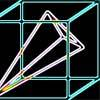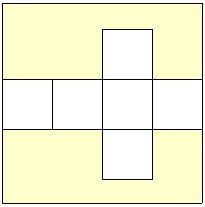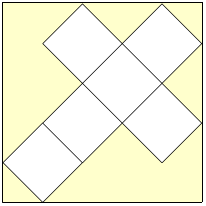#### You may also likeA box of size a cm by b cm by c cm is to be wrapped with a square piece of wrapping paper. Without cutting the paper what is the smallest square this can be?### Plane to See

P is the midpoint of an edge of a cube and Q divides another edge in the ratio 1 to 4. Find the ratio of the volumes of the two pieces of the cube cut by a plane through PQ and a vertex.### Four Points on a Cube

What is the surface area of the tetrahedron with one vertex at O the vertex of a unit cube and the other vertices at the centres of the faces of the cube not containing O?

# Classic Cube

##### Age 16 to 18Challenge Level

I have a large square piece of card 100cm along each side and have decided to make a large cube from it.

I could cut the net of the cube so that its sides are parallel to the sides of the card and, ignoring any tabs needed to join the edges together, it could look like this:
#####What is the volume of this cube?
However, I am wasting a lot of the card by cutting the net in this way and I think I can create a cube with a larger volume if I place a net diagonally on the card, like this:
#####What are the dimensions of the cube with greatest volume I can cut from the card by using different nets and aligning them at an angle to the sides of the card?